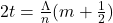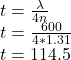Question

. A thin layer of a transparent material with an index of refraction of 1.31 is used as a non-reflective coating on a surface of glass with an index of refraction of 1.43. What should the minimum thickness of the material be for it to be

1.The minimum thickness of the material is 114.5 nm

Explanation:

The remaining part of the question is as follows

What should the minimum thickness of the material be for it to be nonreflecting for light of wavelength 600 nm (in a vacuum)? Please give details of your reasoning.

Both reflections occur against a surface of higher index of refraction, so there is a phase shift at each reflection. The phase shifts cancel each other out, so if we want the emerging rays to be out of phase, we must have

Given

Index of refraction = 1.31

Index of refraction = 1.43

As we knowHere t is the thicknessrepresents the phase shift

n is the refraction index

Substituting the given values we getnm